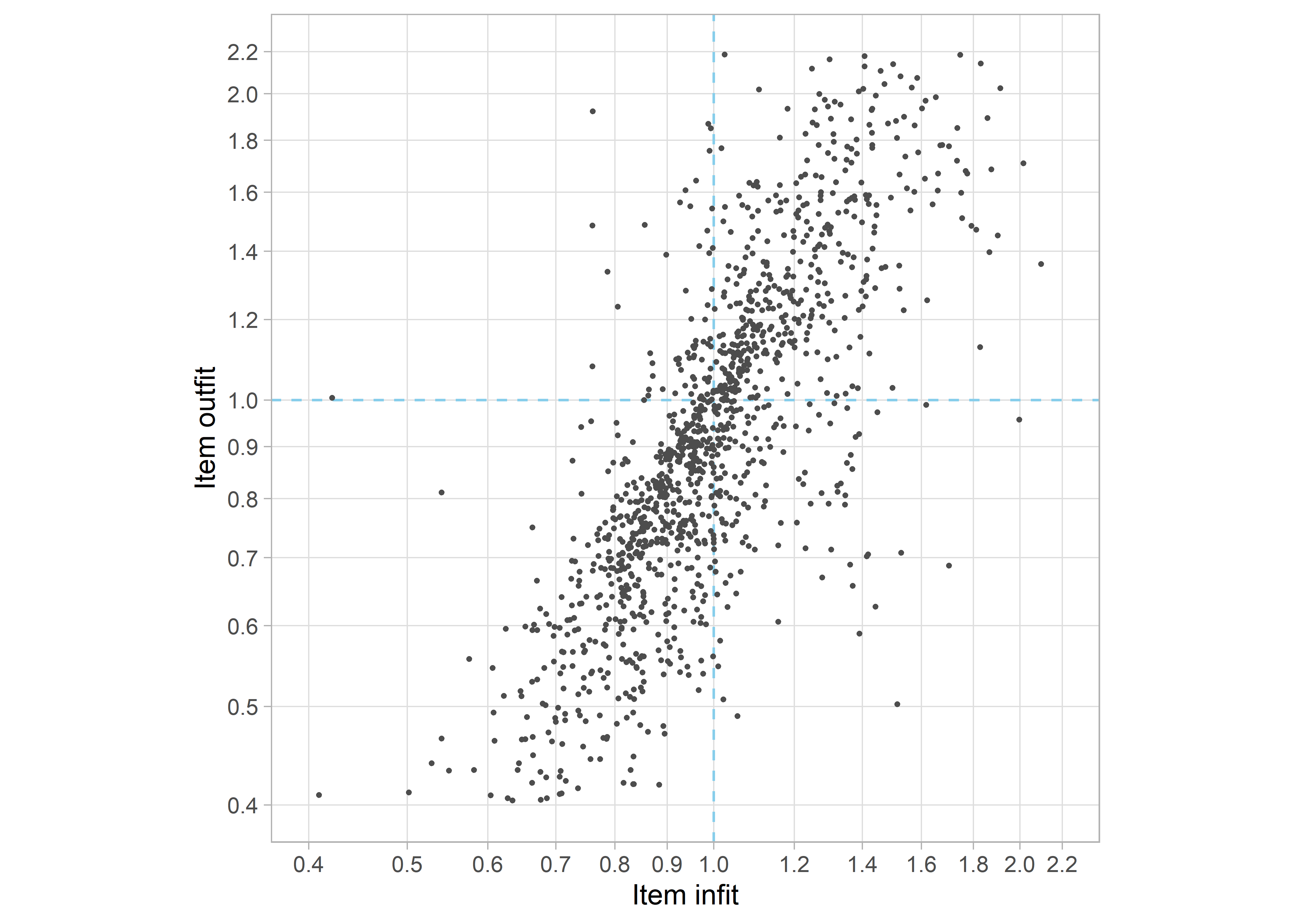## 5.6 Milestone selectionFigure 5.5: Infit and outfit of 1339 items in model 1339_11. About 8 percent of the points falls outside the plot.

Item infit and outfit are convenient statistics for selecting the milestones that fit the model. Figure 5.5 displays the infit and outfit statistics of model 1339_11. The correlation between infit and outfit is high ($$r = 0.84$$). The expected value of the infit and outfit statistics for a perfect fit is 1.0. The centre of infit and outfit in Figure 5.5 is approximately 1.0, so on average one could say the items fit the model. Note however that fit values above and below the values of 1.0 are qualitatively different. Item with fit statistics exceeding 1.0 fit the model less well than expected (underfit), whereas items with fit statistics lower than 1.0 fit the model better than expected (overfit). See Chapter 1, Section 6.1 for more details.

Some practitioners remove both underfitting and overfitting items. However, we like to preserve overfitting items and be more strict in removing items that underfit. The idea is that preservation of the best fitting items may increase scale length, and hence reliability and measurement precision. Figure 5.5 draws two cut-off lines at 1.0. Taking items with infit < 1.0 and outfit < 1.0 will select 631 out of 1339 items for further modelling.

A practical problem of item removal is that it also affects equate group composition. By default, a removed item will also be removed from the equate group, so item removal may reduce the size of an equate group below two items. For passive equates this is no problem, since passive equates do no affect the estimates. However, removal of an underfitting item from an active equate group will break the bridge between the instrument it pertains to and the rest of the item set. Potentially this can result in substantial effects on the D-score distribution of the cohort, as demonstrated in Figure 5.2. As a solution, we force any items that are members of active equate groups to remain in the analysis. If that leads to substantially worse equate fit in the next model, we must search for alternative equate groups that bridge the same instruments and that are less sensitive to misfit.